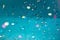# Better JavaScript — Defining FunctionsPhoto by Wonderlane on Unsplash

Like any kind of apps, JavaScript apps also have to be written well.

Otherwise, we run into all kinds of issues later on.

In this article, we’ll look at ways to improve our JavaScript code.

# Use Immediately Invoked Function Expressions to Create Local Scope

Immediately Invoked Function Expressions or IIFEs for short, are functions that are created and called immediately.

For instance, we can create one by writing:

`(function(){})();`

We create the function and we can put whatever want to keep private inside.

It was used to get around JavaScript’s lack of block-scoped variables before ES6.

But now we need this less because we have modules to keep things private.

And we can define block-scoped variables with `let` and `const` .

If we use it in a block, then we may see some issues.

For instance, if we have a loop like:

`function foo(a) {  var result = [];  for (var i = 0; i < a.length; i++) {    (function(j) {      result[i] = () => a[j]    })(i);  }  return result;}`

Then we can’t use `break` or `continue` in the IIFE.

The `this` inside the IIFE would also change because of the traditional function used for the IIFE.

So we can do away with the IIFE, we change the `var` s th `let` and remove the IIFE:

`function wrapElements(a) {  let result = [];  for (let i = 0; i < a.length; i++) {    result[i] = () => a[i]  }  return result;}`

Since `let` is block-scoped the value of `i` will update and passed into the arrow function we set for `result[i]` in each iteration.

# Unportable Scoping of Named Function Expressions

The meaning of the function can change depending on the context even though the function's code looks the same.

For instance, if we have:

`function times(x) {  return x * 5;}`

then that’s a function declaration.

On the other hand, if we have:

`const times = function(x) {  return x * 5;}`

then that’s a function expression.

A function expression is where we define with the `function` keyword and didn’t assign that to a variable.

If we assign that to a variable, then it becomes a function expression.

We can also write:

`const f = function times(x) {  return x * 5;}`

to define a function expression

The difference between anonymous and named function expression is that the latter binds its name to the local variable within a function.

For instance, we can write:

`const traverse = function find(tree, key) {  if (!tree) {    return null;  }  if (tree.key === key) {    return tree.value;  }  return find(tree.left, key) ||    find(tree.right, key);};`

We call `find` in the `find` function.

But when we call it outside, we call it with `traverse` .

Since we can just call `traverse` to do the same thing, having a name in the function’s scope isn’t very useful.

We can also use a function declaration like:

`function find(tree, key) {  if (!tree) {    return null;  }  if (tree.key === key) {    return tree.value;  }  return find(tree.left, key) ||    find(tree.right, key);}`

However, named function expressions are useful for debugging.,

Most modern JavaScript environments produce stack traces with `Error` instances and the name will be used for the stack trace.Photo by Jason Leung on Unsplash

# Conclusion

IIFEs have some uses but not as much as before.

Also, there’re several ways to define a function and they do slightly different things.

Web developer. Subscribe to my email list now at https://thewebdev.info/subscribe/. Email me at hohanga@gmail.com

Web developer. Subscribe to my email list now at https://thewebdev.info/subscribe/. Email me at hohanga@gmail.com